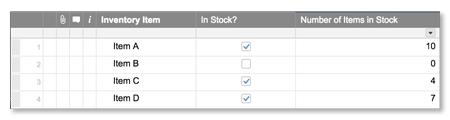# VLOOKUP Function

Looks up a value and returns a corresponding value in the same row but from a different column.
Sample Usage
Syntax
VLOOKUP(
• search_value
• lookup_table
• column_num
• [
match_type
]
)
• search_value
The value to search for, which must be in the first column of lookup_table.
• lookup_table
The cell range in which to search, containing both the search_value (in the leftmost column) and the return value.
• column_num
A number representing the column position (in lookup_table) of the value to return, with the leftmost column of lookup_table at position 1.
• match_type
—[optional]
The default is true. Specifies whether to find an exact match (false) or an approximate match (true).
Usage Notes

Use a VLOOKUP formula to automatically bring in associated content based on criteria in your sheet. For example, bring in someone's role using their name as the criteria.

• You can use VLOOKUP to look up a value from a table in another sheet. For details on referencing cells from other sheets, see Formulas: Reference Data from Other Sheets.
• If VLOOKUP doesn't find a result, you'll receive a #NO MATCH error. Details on troubleshooting this can be found in the Formula Error Messages article.
• You'll receive a #NO MATCH error if there is not a number within the range that is greater than or equal to the search_value.
• If lookup_table is not sorted in ascending order by the first column, then VLOOKUP returns incorrect results.
• The search_value must be in the leftmost column (position 1) of lookup_table.
• To look up text strings, you must enclose the lookup value in quotation marks (for example, “Task E”).
• With the match_type argument:
• Set match_type to false if your lookup_table is not sorted.
• True (the default value) assumes that the range is sorted ascending and returns the nearest match that is less than or equal to ( <= ) search_value.
• False returns the first exact match.
• You can insert the column number into a formula to indicate which column you are retrieving the value from.

For example, the formula =VLOOKUP([Inventory Item]3, [Inventory Item]1:[Number of Items in Stock]4, 3, false) will return the value 4. The formula is written to retrieve a value from the third column (Number of Items in Stock) in the table below.Examples

This example references the following sheet information:

Clothing Item

Units Sold

Price Per Unit

In Stock?

Status

Assigned To

1

T-Shirt

78

\$15.00

true

Green

sally@domain.com

2

Pants

42

\$35.50

false

Red

tim@domain.com

3

Jacket

217

\$200.00

true

Yellow

corey@domain.com

Given the table above, here are some examples of using VLOOKUP in a sheet:

Formula

Description

Result

IF([In Stock?]1 = 1 (true), VLOOKUP("T-Shirt", [Clothing Item]1:Status3, 5))

Return the Status Color. If the “In Stock” column equals 1 (true) look up the value “T-Shirt” in the “Clothing Item” column and produce the value of the “Status Column”, (fourth column in the table).

Green

IF([In Stock?]2 = 0 (false), VLOOKUP([Row #]1, [Row #]1:[In Stock?]3, 2))

Return Item Out of Stock. If the “In Stock” column equals 0 (false) look up the value of Row 2 and produce the value of the Clothing Item, column 2

Pants

VLOOKUP("Jacket", [Clothing Item]1:[Price Per Unit]3, 3, false) * [Units Sold]3

Return total revenue. Look up the value “Jacket” in the “Clothing Item” column, if found, produce the value in the “Price Per Unit” column (\$200). Then multiple this by the “Units Sold” column value (217).

43400

VLOOKUP([Clothing Item]1, {Range on Reference Sheet}, 2, false)

Return the assigned to contact email. Look up the value in the “Clothing Item” column row 1 on the reference sheet, if found, produce, produce the value in the Assigned To column (column 2) on the reference sheet.

sally@domain.com

#### Still need help?

Use the Formula Handbook template to find more support resources, and view 100+ formulas, including a glossary of every function that you can practice working with in real time, and examples of commonly used and advanced formulas.

Find examples of how other Smartsheet customers use this function or ask about your specific use case in the Smartsheet online Community.